# Electromagnetic waves and circular dichroism: an animated tutorial

By Andras Szilagyi (szilagyi (dot) andras (at) ttk (dot) mta (dot) hu). Comments and suggestions welcome!Auf Deutsch |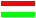Magyarul

 Note: If you find the animations presented on the following pages useful, try EMANIM, my educational web app to create rotatable, zoomable 3D visualizations of electromagnetic waves with interactively variable parameters!

# I. Basic concepts: Electromagnetic waves and types of polarization

Electromagnetic waves are periodic changes of electric and magnetic fields in space and time. They propagate at the speed of light. At any point of a light beam, the magnetic field is always perpendicular to the electric field. Therefore, for the sake of clarity, only the electric field is shown in the animations here. Both the electric and magnetic fields oscillate in a plane perpendicular to the direction of propagation of the light beam.

In the following movies, a wave propagating in space is visualized. The light beam is intersected at a fixed point of space by a plane perpendicular to the direction of propagation of the light beam. At the point of intersection, the electric field is measured and shown as a vector in the pictures. In other words, the length and direction of the vector represents the strength and the direction of the electric field measured at the starting point of the vector. Don't interpret these pictures as showing waves located in space! The light beam is not spread laterally.

# I/1. Plane-polarized waves 1.

If the vector of the electric field (measured at a fixed point of space) oscillates along a straight line then the waves are called plane-polarized or linearly polarized waves. The following animation presents a wave that is plane-polarized in a vertical plane.

 3D view Front view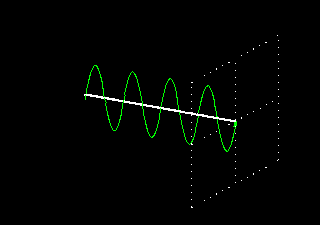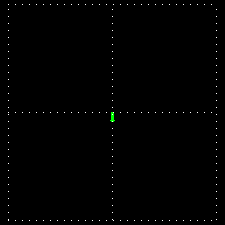# I/2. Plane-polarized waves 2.

The following animation presents a wave that is plane-polarized in a horizontal plane.

 3D view Front view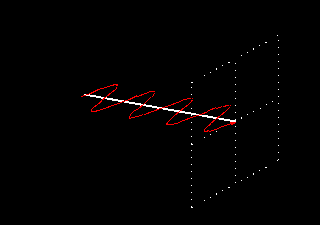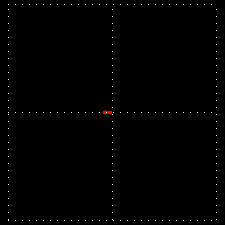# I/3. Superposition of plane-polarized waves 1.

When two electromagnetic waves plane-polarized in two perpendicular planes are present simultaneously then the electric fields are added according to the rules of vector addition, a.k.a. 'parallelogram rule' (superposition). The properties of the resulting electromagnetic wave depend on the intensities and phase difference of the component waves.

The following animation presents the superposition of two waves that have the same amplitude and wavelength, are polarized in two perpendicular planes and oscillate in the same phase. Oscillating in the same phase means that the two waves reach their peaks and cross the zero line in the same moments. The superposing components are visualized with red and green color, respectively. The wave resulting from the superposition is shown in light blue.

 3D view Front view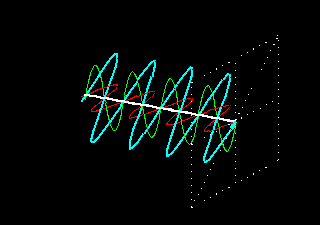# I/4. Superposition of plane-polarized waves 2.

When two waves plane-polarized in two perpendicular planes meet out of phase then the wave resulting from the superposition of the two waves will no longer be plane-polarized.

The following animation presents the superposition of two waves that have the same amplitude and wavelength and are polarized in two perpendicular planes but there is a phase difference of 90 degrees between them. A phase difference of 90° means that when one wave is at its peak then the other one is just crossing the zero line. The superposing components are visualized with red and green colors and the wave resulting from the superposition is shown in light blue.

 3D view Front view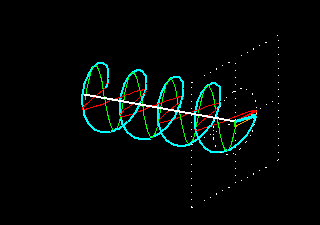As you can see, the result of the superposition is a special electromagnetic wave. At any fixed point in space that is in the line of the propagation of this wave, the electric field vector rotates in a circle while its length remains constant. Such waves are called circularly polarized waves. As the 3D picture shows, a circularly polarized wave can be visualized with a spiral line; the wave propagates as a function describing a spiral instead of one describing a sine curve.

In this picture, the electric field vector rotates clockwise when looked at from the direction of propagation.

# I/5. Superposition of plane-polarized waves 3.

The following animation shows what happens when the two waves shown on the previous page are added with a phase difference of -90 degrees instead of 90 degrees. This requires shifting of one wave relative to the other one along their axis so that there is a 3/4 wavelength difference between them.

 3D view Front view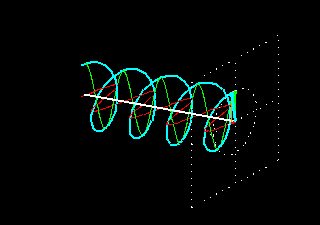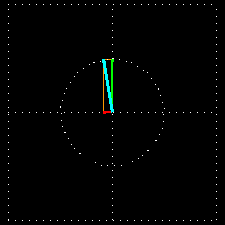As you can see, again a circularly polarized light results but the electric field vector of this light rotates counterclockwise, rather than clockwise, when looked at from the direction of propagation. If you compare the spiral line representing the wave with the spiral line on the previous page, you will observe that one of them is a left-handed spiral and the other one is a right-handed one.

Based on this, we can distinguish right and left circularly polarized lights.

# I/6. Circularly polarized waves

On this page, the animations presenting the two types of circularly polarized light are shown together so that you can compare them more easily.When the intersecting planes are looked at from the front then the following images are seen:# I/7. Superposition of circularly polarized waves

As we have seen in the previous pages, two plane-polarized waves can meet. Of course, two circularly polarized waves can meet as well. In that case, the fields are added according to the rules of vector addition, just as with plane-polarized waves.

The superposition of two circularly polarized light beams can result in various outcomes. The most interesting result is obtained when a left circularly polarized wave and a right circularly polarized wave are added. Let's assume that the two light beams have equal amplitudes and wavelengths. The following animation shows this superposition and the resulting wave (red and green colors indicate the two superposing components and light blue indicates the resulting wave):

 3D view Front view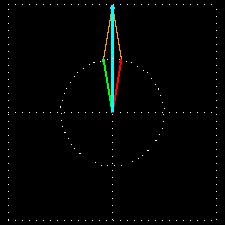As we see, the result of superposing two circularly polarized waves is a plane-polarized wave.

By thinking "backwards", we can reach the following very important conclusion: Any linearly polarized light wave can be obtained as a superposition of a left circularly polarized and a right circularly polarized light wave, whose amplitudes are identical.

# II. Interaction of light and matter

If light enters matter, its properties may change. Namely, its intensity (amplitude), polarization, velocity, wavelength, etc. may alter. The two basic phenomena of the interaction of light and matter are absorption (or extinction) and a decrease in velocity.

Absorption means that the intensity (amplitude) of light decreases in matter because matter absorbs a part of the light. (Intensity is the square of amplitude.)

The decrease in velocity (i.e. the slowdown) of light in matter is caused by the fact that all materials (even materials that do not absorb light at all) have a refraction index, which means that the velocity of light is smaller in them than in vacuum. The refraction index is the ratio of the velocities of light measured in vacuum and in the given material.

The following animations present these basic phenomena.

# II/1. Plane-polarized waves in an absorbing medium

The following animation shows what happens when a plane-polarized wave traverses a medium that absorbs light but does not refract it (its refraction index is 1). A piece of material is shown, with an intersecting plane both in front and behind it. We measure the field vector where the light beam intersects these two intersecting planes.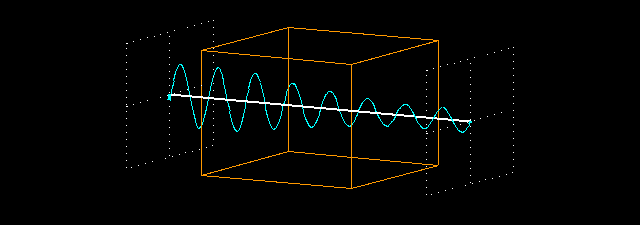The following animation shows the intersecting planes looked at from the front. On the left, the light beam in its original state; on the right, the light beam after crossing the piece of material.As you can see, when the light enters the medium, it becomes fainter, i.e. its intensity decreases according to an exponential function inside the medium. After exiting the material, the field vector oscillates the same way as before entering it but its amplitude is only about 36% of the earlier value.

# II/2. Circularly polarized waves in an absorbing medium

The following animation shows what happens when a circularly polarized light wave traverses a light-absorbing medium (the refraction index is assumed to be 1, as before). We measure the field vector of the light before it enters and after it exits the medium, just like on the previous page.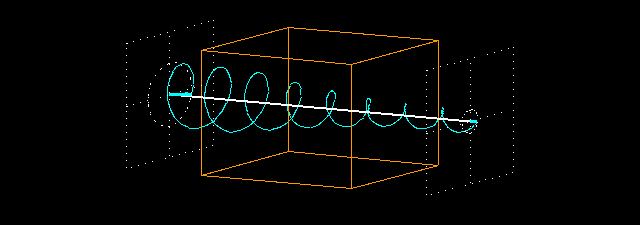The following animation shows the two intersecting planes from the front. On the left, before entering the material, on the right, after exiting the material.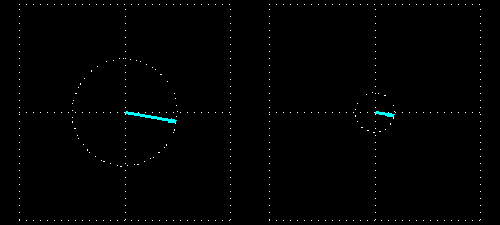As you can see, the intensity of light decreases exponentially inside the shown piece of material. After the light exits the medium, its field vector rotates as before but its length is only about 36% of the original value.

# II/4. Plane-polarized waves in a refracting medium

The following animation shows what happens when a plane-polarized wave traverses a non-absorbing medium with a refraction index greater than 1.0. We measure the field vector of the light wave before its entry into the medium and after its exit, just like on the previous page.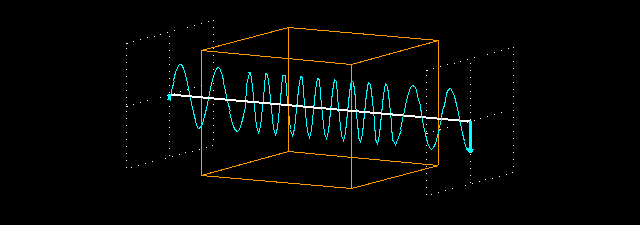The following animation shows the two intersecting planes from the front. On the left, the field vector before the light enters the material; on the right, after the light exits the material.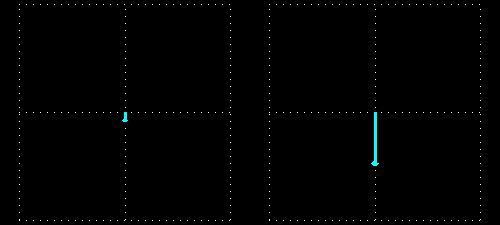When the light beam enters the piece of material, it slows down because the refracting index of the material is greater than 1.0. Its frequency does not change, therefore its wavelength decreases (the product of the frequency and the wavelength should be equal to the velocity of light). In these animations, we used a refraction index n=2.2. This means that the velocity of light in the medium is 1/2.2 times the velocity of light in vacuum, and its wavelength also decreases to 1/2.2 times the original value. When the light exits the material, its velocity and wavelength are restored to their original (vacuum) values. Since the material does not absorb light, the intensity of the light does not decrease.

The distance between the intersecting planes in front of and behind the piece of material is exactly 8 times the vacuum wavelength of the light. Therefore, if the piece of material is not present, the field vectors at the two intersecting planes oscillate in exactly the same way: they are in phase. But if the piece of material is placed between the two intersecting planes, the light slows down in the material and it has 8.8 full periods inside the material, instead of 4 periods. So there are 12.8 periods between the two intersecting planes (there are 2 periods before the piece of material and 2 periods after it). Because this is not an integral number, the two field vectors at the intersecting planes do not oscillate in the same phase any longer.

# II/5. Circularly polarized waves in a refracting medium

The following animation shows what happens when a circularly polarized wave traverses a non-absorbing medium with a refraction index greater than 1.0. We measure the field vector before and after the piece of material, just like on the previous page.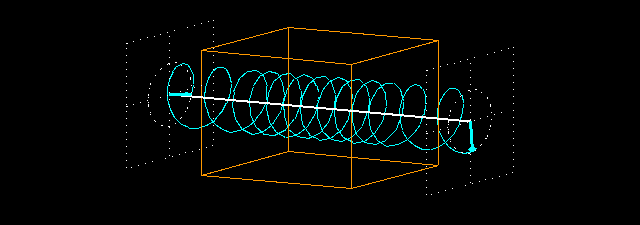The following animation shows the two intersecting planes from the front. On the left, before the material, on the right, after the material.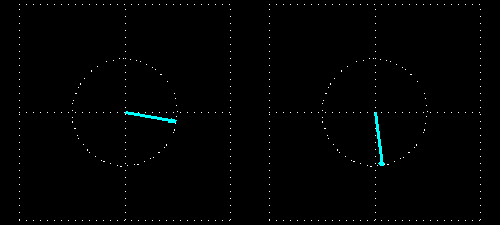The phenomenon seen here is very similar to that presented on the previous page: the wavelength is much smaller in the medium than before and after it because the light is slower in the medium. The intensity of the light does not decrease: the field vector of the exiting light is of the same length as that of the entering light because there is no absorption.

But the phase of the wave changes here again. If the piece of material was not present then the field vectors at the two intersecting planes would rotate exactly in the same phase because their distance is an integral multiple of the wavelength. This situation changes when we put the piece of material in between the two intersecting planes: the light slows down in the piece of material (whose thickness is 4 times the wavelength), and it makes 8.8 full periods inside the material instead of 4 full periods. This results in a 72° phase difference between the entering and exiting light waves. This is clearly seen in the animations showing the intersecting planes.

Based on the presented animations, it is not hard to imagine what happens when the light wave traverses a medium that absorbs AND refracts the light at the same time. In that case, the length of the field vector (amplitude) of the light exiting the material decreases and its phase changes as well in comparison with the amplitude and phase of the light entering the material. We do not present these cases on animations but we encourage the reader to imagine them.

# II/6. Plane-polarized waves in a medium with circular dichroism

Some materials possess a special property: they absorb left circularly polarized light to a different extent than right circularly polarized light. This phenomenon is called circular dichroism.

As demonstrated, any linearly polarized light can be obtained as the superposition of a left circularly polarized and a right circularly polarized light wave. Therefore, if linearly polarized light traverses a medium that shows circular dichroism, its properties will change because the medium absorbs the two circularly polarized components to a different extent.

The following animation shows what happens when a plane-polarized (the plane of polarization is vertical here) light wave (indicated by light blue color) traverses a medium that does not absorb the left circularly polarized component of the wave at all (this is the circular wave shown in red) but highly absorbs the right circularly polarized component (this is the circular wave shown in green).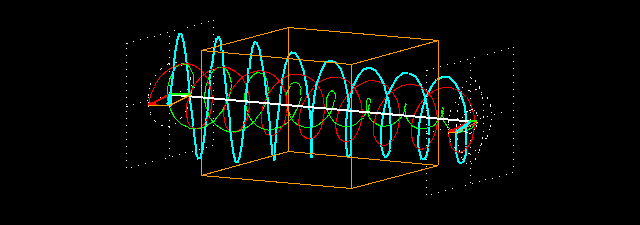We placed an intersecting plane before and after the piece of material and we show the field vectors at the point of intersection of the light beam and the intersecting planes. The following animation shows the intersecting planes from the front. On the left, the plane before the material; on the right, the plane after the material.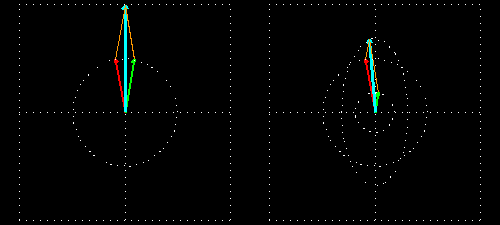So what is here? The component shown in red traverses the medium unchanged and the component shown in green gets fainter: its intensity decreases to about 36% of the original value. The superposition of the two components is no longer a linearly polarized wave: the resulting field vector does not oscillate along a straight line but it rotates along and ellipsoid path. Such a light wave is called an elliptically polarized light.

Take a close look and notice that the big axis of the ellipse is parallel to the polarization plane of the original light wave. This is always the case, regardless of which circular component is absorbed more by the medium. But the direction of rotation of the elliptically polarized light (or, more exactly, its field vector) is determined by the circular component that remains stronger after traversing the material. In our case, the field vector of the elliptically polarized light rotates in the same direction as that of the component shown in red color, which traverses the medium without losing intensity.

Of course, it is very unusual for a material not to absorb one circular component at all. Real materials usually absorb both components, to a different extent. It was only for the sake of simplicity that we presented a situation where the material is completely transparent to one of the circularly polarized components.

How elliptical the plane-polarized wave becomes after traversing the medium is determined by the difference between the absorptions of the two circularly polarized components. In the most extreme case, the material almost completely extincts one component--and then the plane-polarized wave almost becomes a perfect circularly polarized light because the other circular component disappears.

Please note: circular dichroism makes plane-polarized light elliptically polar.

Notice: The word "dichroism" means that the material absorbs two different types of light differently. If the adjective "circular" does not precede it then it refers to linearly polarized light: simple dichroism is when a material absorbs light polarized in some plane differently than light polarized in a plane perpendicular to it. Materials having high dichroism can be used as polar filters because they are practically transparent to light polarized in one direction but practically opaque to light polarized in the perpendicular direction.

# II/7. Plane-polarized waves in a medium with circular birefringence

There are materials having another special property: their refraction index is different for left and right circularly polarized light. This phenomenon is called circular birefringence.

As demonstrated, any linearly polarized light can be obtained as the superposition of a left circularly polarized and a right circularly polarized light wave. Therefore, if linearly polarized light traverses a medium that shows circular birefringence, its properties will change because the the two circularly polarized components slow down in the medium to a different extent.

The following animation shows what happens when a plane-polarized (the plane of polarization is vertical here) light wave (indicated by light blue color) traverses a medium that does not slow down the left circularly polarized component (this is the circular wave shown in red) of the wave at all but slows down the right circularly polarized component (this is the circular wave shown in green) somewhat. For the latter component, the refraction index of the material is n=1.05.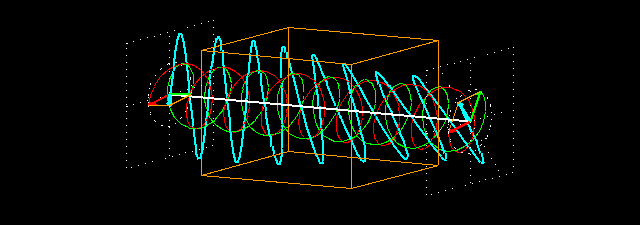We placed an intersecting plane before and after the piece of material and we show the field vectors at the point of intersection of the light beam and the intersecting planes. The following animation shows the intersecting planes from the front. On the left, the plane before the material; on the right, the plane after the material.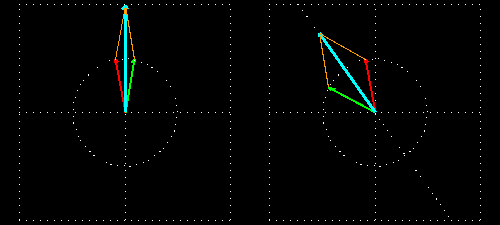As you can see, the exiting wave continues to be a plane-polarized wave but its plane of polarization is no longer vertical; the polarization plane has rotated by 36 degrees.

What is the reason for that? The component shown in red traverses the medium unchanged while the component shown in green gets slower and its wavelength decreases in the medium. This slowdown and the decreased wavelength is hard to see in the figure because the refraction index (1.05) is close to 1.0 (we did not use a greater refraction index here because that would result in a confusing image). But as a result of this refraction index, the wave shown in green makes 4.2 full periods in the medium instead of 4 full periods, therefore its field vector will be 72° further on its rotating path than if the medium were not present.

And what happens to the sum of the two circular components? Before the medium, the field vectors of the components coincide when they point vertically up or down. But after the light exits the medium, this situation changes. When the red component points vertically up, the green component makes a 72-degree angle with the vertical direction. Their vectorial sum slants 36 degrees from the vertical direction. The superposition of the two circularly polarized components is a plane-polarized wave with a plane of polarization that is rotated by 36° with respect to the original polarization plane.

Of course, it is very unusual for a material to have a refraction index of 1.0 for one circularly polarized component. Real materials usually have refraction indices greater than 1.0 (but not equal) for both circular components. It was only for the sake of simplicity that we presented a situation where one component is not slowed down by the material at all.

The angle by which the polarization plane of the light exiting the medium rotates with respect to the original polarization plane is determined by the difference between the refraction indices for the two circularly polarized components (and of course on the length of path traversed in the medium). If the difference between the refraction indices is large enough and the piece of material is thick enough then the plane of polarization can make several full rotations as light traverses the material.

Please note: circular birefringence rotates the plane of polarization of plane-polarized light.

Materials that rotate the polarization plane of the light traversing them (this is optical rotation) or make plane-polarized light elliptically polar are called optically active materials. As we can see, optical activity is caused by circular birefringence or circular dichroism.

Notice: The word "birefringence" means double refraction (bi-refringence). If the adjective "circular" does not precede it then it refers to those materials (crystals) whose refraction index depends on the direction of the light. Crystals showing birefringence have a number of interesting properties which we do not discuss here.

# II/8. Plane-polarized waves in a medium with both circular dichroism and circular birefringence

In reality, it rarely occurs that a material exhibits circular dichroism but no circular birefringence or it exhibits circular birefringence but no circular dichroism with respect to light of a certain wavelength.

The following animation shows what happens when a plane-polarized wave (shown in light blue) traverses a medium having both circular dichroism and circular birefringence. Just like on the previous two pages, the two circularly polarized components of the plane-polarized light are shown in red and green color, respectively. The red component traverses the medium unchanged, but the medium has absorption and a refraction index with respect to the green component.We placed an intersecting plane before and after the piece of material and we show the field vectors at the point of intersection of the light beam and the intersecting planes. The following animation shows the intersecting planes from the front. On the left, the plane before the material; on the right, the plane after the material.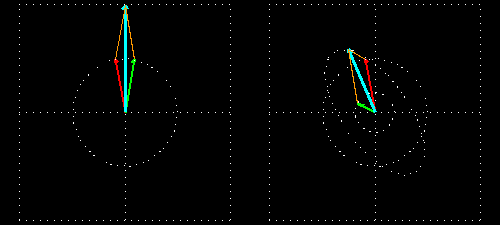As you could suspect from the phenomena shown on the previous pages, the incident light suffers two modifications here: because of the circular dichroism, it becomes elliptical; and because of the circular birefringence, its polarization gets rotated. Since the exiting light is no longer plane-polarized, it is not the plane of polarization that gets rotated but the big axis of the ellipse of polarization of the elliptically polarized light.

The ellipticity of the light exiting the medium is determined by the difference between the absorptions with respect to the left and right circularly polarized components. The angle made by the big axis of the ellipse with respect to the original polarization plane is determined by the difference between the refraction indices with respect to the left and right circularly polarized components.

With the appropriate instrument, the ellipticity and the angle of rotation of the polarization plane of light can be measured. From those data, the difference between absorptions and refraction indices with respect to left and right circularly polarized lights can be calculated.

Circular dichroism and circular birefringence are caused by the asymmetry of the molecular structure of matter. The optical activity of solutions of biological macromolecules provides information about the structural properties of the macromolecules. This is discussed in more detail in the literature about the spectroscopy of biological macromolecules.

## The End!

Last page: How were these animations produced?

# How were these animations produced?

The animations were made with version 3.7 of the gnuplot graphing program, by giving the appropriate mathematical functions. We used the program under the Linux operating system with Xwindows graphical interface. We created the GIF image files showing individual phases of the animations using the import command in Linux, which makes a snapshot of the gnuplot window and saves it in a file. The individual frames of the animations were put together in animated GIF files using version 1.11.1 of the gifsicle program for making, manipulating and optimizing animated GIF files. This program was used under Linux as well.

The animations were made by Andras Szilagyi, who welcomes suggestions and questions (e-mail: szilagyi (dot) andras (at) ttk (dot) mta (dot) hu).

January 30, 2002. (Slideshow prepared in 2014 using Slideous.)

of {\$slidecount}